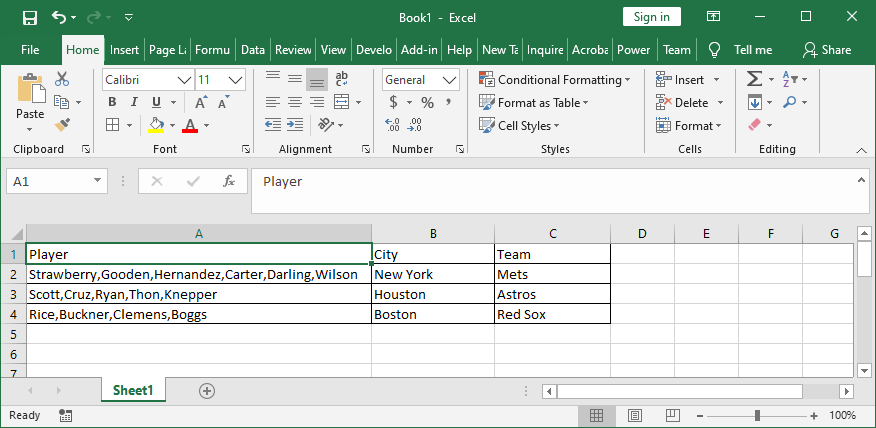Search

# Splitting Delimited Text Inside Cells Onto New Rows

The vba code shown below posted here, can be used to split text entered with delimiters inside a cell onto separate rows and repeat the information from adjacent columns on the new rows. So, in this example we have the names of baseball players separated with commas in column A, and the city and team they played for in columns B and C.When the macro is run the names of each player are listed on separate rows, and the name of their city and team repeats in column B and C.Edit the line "With Range("A1:C" & LR)" from C to a higher column letter to account for longer arrays. Change given delimiters on these lines:

If InStr(.Value, ",") = 0 Then

X = Split(.Value, ",")Sub Splt() Dim LR As Long, i As Long Dim X As Variant Application.ScreenUpdating = False LR = Range("A" & Rows.Count).End(xlUp).Row Columns("A").Insert For i = LR To 1 Step -1 With Range("B" & i) If InStr(.Value, ",") = 0 Then .Offset(, -1).Value = .Value Else X = Split(.Value, ",") .Offset(1).Resize(UBound(X)).EntireRow.Insert .Offset(, -1).Resize(UBound(X) - LBound(X) + 1).Value = Application.Transpose(X) End If End With Next i Columns("B").Delete LR = Range("A" & Rows.Count).End(xlUp).Row With Range("A1:C" & LR) On Error Resume Next .SpecialCells(xlCellTypeBlanks).FormulaR1C1 = "=R[-1]C" On Error GoTo 0 .Value = .Value End With Application.ScreenUpdating = True End Sub